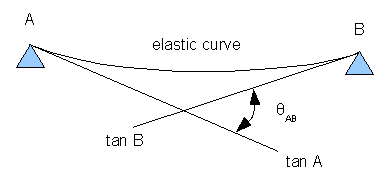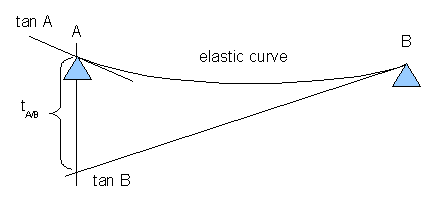# Moment area method

Method for finding deflections in a framed structure by the use of moment area curve.

## First TheoremTheorem 1: The change in slope between any two points on the elastic curve equals the area of the M/EI diagram between these two points.

$\theta_{AB} = {\int_A}^B \frac{M}{EI}\;dx$

where

• M moment
• EI flexural rigidity
• $\theta_{AB}$ ... change in slope between points A and B
• A, B ... points on the elastic curve

## Second TheoremTheorem 2: The deviation of the tangent at point B on the elastic curve with respect to the tangent at point A equals the "moment" of the M/EI diagram between points A and B computed about point A (the point on the elastic curve), where the deviation $t_{A/B}$ is to be determined.

$t_{A/B} = {\int_A}^B \frac{M}{EI} \bar{x} \;dx$

where

• M moment
• EI flexural rigidity
• $t_{A/B}$ ... deviation of tangent at point B with respect to the tangent at point A
• $\bar{x}$ ... centroid of M/EI diagram measured horizontally from point A
• A, B ... points on the elastic curve

## References

• Russel C. Hibbeler: Structural Analysis, 3rd Edition, Prentice Hall, 1995, chapter 8, p. 354-569, ISBN 0-02-354041-9